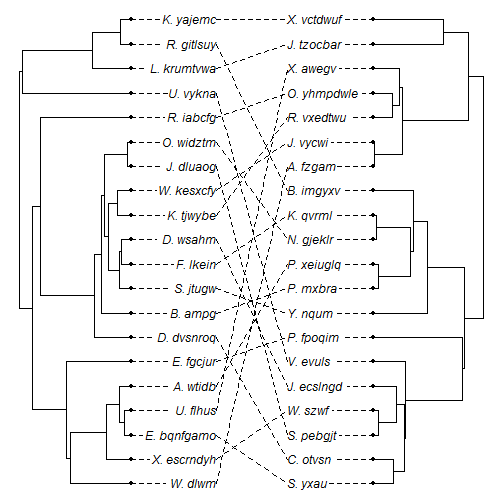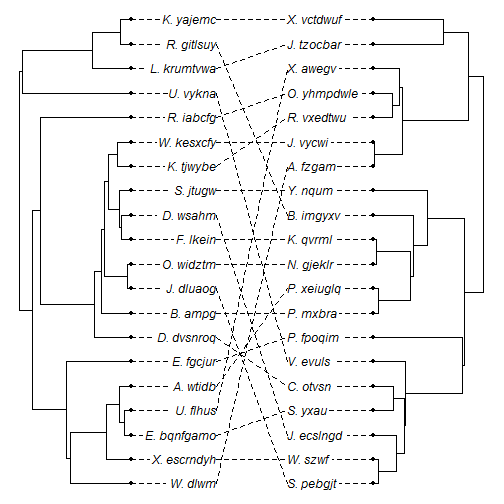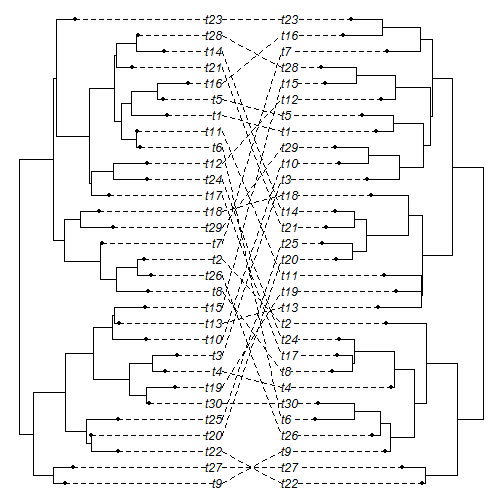## Sunday, July 12, 2015

### New method for "co-phylogenetic" plotting

I just added a function to plot co-phylogenetic trees (that is, trees which have been plotted to maximize the vertical alignment of tips). This is not meant to displace or replace the function `cophyloplot` from the ape package, just as an alternative. It also does not (yet) contain the full range of plotting options that can be accesses using (for example) `plot.phylo` or `plotSimmap` (neither of which are used internally by this function. Consequently, though the function produces a nice visualization (in my opinion) - it is not particularly flexible.

It works in the following way. First, the function `cophylo` takes two trees and an “association” table to (by default) try to rotate all the nodes of the tree so that the match the specified association as closely as possible. It returns an object of class `"cophylo"` which is then plotted using an S3 plotting method that I have written for that object class.

Here is a demo using a simulated tree & association matrix:

``````library(phytools)
source("http://www.phytools.org/cophylo/v0.1/cophylo.R")
N<-20
tips.tr1<-replicate(N,paste(sample(LETTERS,1),"._",
paste(sample(letters,round(runif(n=1,min=4,max=8))),
collapse=""),sep=""))
tips.tr2<-replicate(N,paste(sample(LETTERS,1),"._",
paste(sample(letters,round(runif(n=1,min=4,max=8))),
collapse=""),sep=""))
tr1<-pbtree(n=N,tip.label=tips.tr1)
tr2<-pbtree(n=N,tip.label=sample(tips.tr2))
assoc<-cbind(tips.tr1,tips.tr2)
## here is our association matrix
assoc
``````
``````##       tips.tr1      tips.tr2
##  [1,] "W._dlwm"     "A._fzgam"
##  [2,] "X._escrndyh" "W._szwf"
##  [3,] "E._bqnfgamo" "S._yxau"
##  [4,] "U._flhus"    "X._awegv"
##  [5,] "A._wtidb"    "P._xeiuglq"
##  [6,] "E._fgcjur"   "P._fpoqim"
##  [7,] "D._dvsnroq"  "C._otvsn"
##  [8,] "B._ampg"     "P._mxbra"
##  [9,] "S._jtugw"    "Y._nqum"
## [10,] "F._lkein"    "K._qvrml"
## [11,] "D._wsahm"    "J._ecslngd"
## [12,] "K._tjwybe"   "R._vxedtwu"
## [13,] "W._kesxcfy"  "J._vycwi"
## [14,] "J._dluaog"   "S._pebgjt"
## [15,] "O._widztm"   "N._gjeklr"
## [16,] "R._iabcfg"   "O._yhmpdwle"
## [17,] "U._vykna"    "V._evuls"
## [18,] "L._krumtvwa" "J._tzocbar"
## [19,] "R._gitlsuy"  "B._imgyxv"
## [20,] "K._yajemc"   "X._vctdwuf"
``````

Now let's run our functions. First without rotation, then with rotation (the default):

``````## no rotation
obj<-cophylo(tr1,tr2,rotate=FALSE,assoc=assoc)
obj
``````
``````## Object of class "cophylo" containing:
##
## (1) 2 (possibly rotated) phylogenetic trees in an object of class "multiPhylo".
##
## (2) A table of associations between the tips of both trees.
``````
``````plot(obj)
````````````## with rotation
obj<-cophylo(tr1,tr2,assoc=assoc)
obj
``````
``````## Object of class "cophylo" containing:
##
## (1) 2 (possibly rotated) phylogenetic trees in an object of class "multiPhylo".
##
## (2) A table of associations between the tips of both trees.
``````
``````plot(obj)
``````If we don't specify an association matrix, then the function assumes that the tip labels are meant to match exactly. We also do not need to use trees that are ultrametric. Here is an example that demonstrates both points:

``````tr1<-rtree(n=30)
tr2<-rtree(n=30)
obj<-cophylo(tr1,tr2)
plot(obj)
``````That's it!

1.Here is a much more flexible and versatile version that I just posted.

2.This comment has been removed by the author.

3.Hi Liam, i like this function very much and thanks for your hard work. I want to compare two tree with different tips, it is not problem by using the command

cophyloplot(tr1,tr2,assoc=matrix)

however, 'phylo' come up the following error:

obj<-cophylo(tr1,tr2,assoc=matrix)
Rotating nodes to optimize matching...
Error in if (oo > pp) tree <- tt : missing value where TRUE/FALSE needed

1.PS:
Trees with different numbers of tips and partial matching to each other. In addition, smaller tree match to multiple tips in the bigger tree.
the number of column of matrix( in the 'assoc=matrix') is equal to the number of tips on the smaller tree.

2.Hi. Can you email me (liam.revell@umb.edu) the example? Otherwise it will be hard for me to investigate this issue. - Liam

4.Hi Liam, thank you so much for this blog.
I am using cophylo() function and I wondered which would be the easiest way to obtain the statistical significance of the comparison, like a permutation test or something like that.

Note: due to the very large amount of spam, all comments are now automatically submitted for moderation.